Documentation

# idinput

Generate input signals

## Syntax

``u = idinput(N)``
``u = idinput([N,Nu])``
``u = idinput([Period,Nu,NumPeriod])``
``u = idinput(___,Type)``
``u = idinput(___,Type,Band)``
``u = idinput(___,Type,Band,Range)``
``````[u,freq] = idinput(___,'sine',Band,Range,SineData)``````

## Description

The `idinput` command generates an input signal with specified characteristics for your system. You can use the generated input, and simulate the response of your system to study system behavior. For example, you can study the system response to periodic inputs. The system can be an actual physical system or a model such as a Simulink® model. You can also design optimal experiments. For example, you can determine which input signals isolate faults or nonlinearities in your system. You can also use `idinput` to design an input that has sufficient bandwidth to excite the dynamic range of your system.

example

````u = idinput(N)` returns a single-channel random binary input signal `u` of length `N`. The generated signal values are either -1 or 1.```

example

````u = idinput([N,Nu])` returns an `Nu`-channel random binary input signal, where each channel signal has length `N`. The signals in each channel differ from each other.```

example

````u = idinput([Period,Nu,NumPeriod])` returns an `Nu`-channel periodic random binary input signal with specified period and number of periods. Each input channel signal is of length `NumPeriod`*`Period`.```

example

````u = idinput(___,Type)` specifies the type of input to be generated as one of the following:`'rbs'` — Random binary signal`'rgs'` — Random Gaussian signal`'prbs'` — Pseudorandom binary signal `'sine'` — Sum-of-sinusoids signalUse with any of the previous input argument combinations.```

example

````u = idinput(___,Type,Band)` specifies the frequency band of the signal. For pseudorandom binary signals (PRBS), `Band` specifies the inverse of the clock period of the signal.```

example

````u = idinput(___,Type,Band,Range)` specifies the amplitude-range of the signal.```

example

``````[u,freq] = idinput(___,'sine',Band,Range,SineData)``` specifies the `Type` as a sum-of-sinusoids signal and specifies the characteristics of the sine waves used to generate the signal in `SineData`. You can specify characteristics such as the number of sine waves and their frequency separation. The frequencies of the sine waves are returned in `freq`.```

## Examples

collapse all

Generate a single-channel random binary input signal with 200 samples.

```N = 200; u = idinput(N);```

`u` is a column vector of length 200. The values in `u` are either -1 or 1.

Create an `iddata` object from the generated signal. For this example, specify the sample time as 1 second.

`u = iddata([],u,1);`

To examine the signal, plot it.

`plot(u)`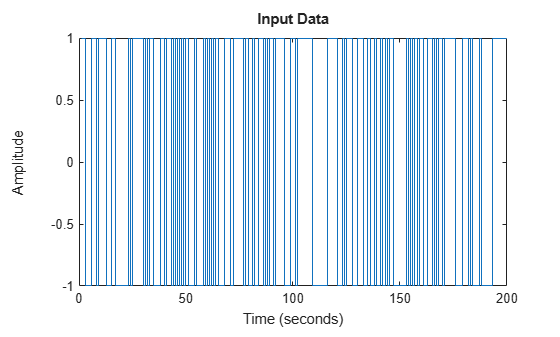The generated signal is a random binary input signal with values -1 or 1. You can use the generated input signal to simulate the output of your system using the `sim` command.

Generate a two-channel random binary input signal with 200 samples.

```N = 200; u = idinput([N,2]);```

`u` is a 200-by-2 matrix with values -1 or 1.

Create an `iddata` object from the generated signal. For this example, specify the sample time as 1 second.

`u = iddata([],u,1);`

Plot the signals for the two channels, and examine the signals.

`plot(u)`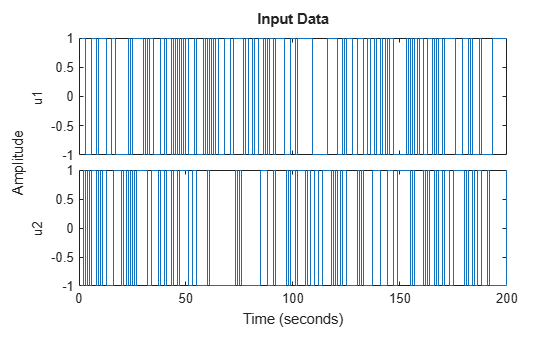The plot shows the two generated random binary signals with values -1 or 1.

Generate a single-channel periodic random binary input signal with a period of 10 samples and 5 periods in the signal.

```NumChannel = 1; Period = 10; NumPeriod = 5; u = idinput([Period,NumChannel,NumPeriod]);```

`u` is a column vector of length 50 (= Period*NumPeriod). The values in `u` are either -1 or 1.

Create an `iddata` object from the generated signal. Specify the sample time as 1 second.

`u = iddata([],u,1);`

Plot the signal.

`plot(u)`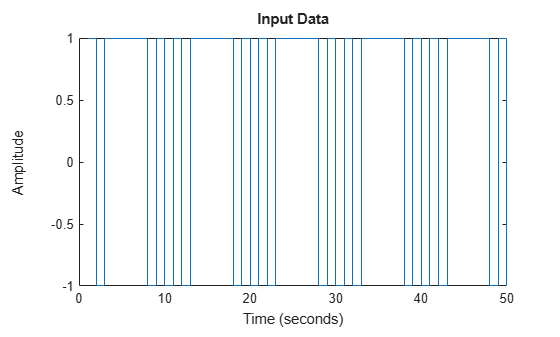As specified, the generated single-channel periodic random binary input signal has a period of 10 seconds, and there are 5 whole periods in the signal.

Generate a single-channel periodic random Gaussian input signal with a period of 50 samples and 5 periods in the signal. First generate the signal using the entire frequency range, then specify a passband.

```NumChannel = 1; Period = 50; NumPeriod = 5; u = idinput([Period,NumChannel,NumPeriod],'rgs');```

`u` is a column vector of length 250 (= Period*NumPeriod).

Create an `iddata` object from the generated signal, and plot the signal. For this example, specify the sample time as 0.01 seconds.

```u = iddata([],u,0.01); plot(u)```The plot shows that `u` contains a random segment of 50 samples, repeated 5 times. The signal is a Gaussian white noise signal with zero mean and variance one.

Since the sample time is 0.01 seconds, the generated signal has a period of 0.5 seconds. The frequency content of the signal spans the entire available range (0-50 Hz).

Now specify a passband between 0 and 25 Hz ( = 0.5 times the Nyquist frequency).

```Band = [0 0.5]; u2 = idinput([Period,NumChannel,NumPeriod],'rgs',Band);```

Create an `iddata` object, and plot the signal.

```u2 = iddata([],u2,0.01); plot(u2)```The frequency content of the generated signal `u2` is limited to 0-25 Hz.

A pseudorandom binary input signal (PRBS) is a deterministic signal whose frequency properties mimic white noise. A PRBS is inherently periodic with a maximum period length of ${2}^{n}-1$, where integer n is the order of the PRBS. For more information, see Pseudorandom Binary Signals.

Specify that the single-channel PRBS value switches between -2 and 2.

`Range = [-2,2];`

Specify the clock period of the signal as 1 sample. That is, the signal value can change at each time step. For PRBS signals, the clock period is specified in `Band` = [0 B], where `B` is the inverse of the required clock period.

`Band = [0 1];`

Generate a nonperiodic PRBS of length 100 samples.

`u = idinput(100,'prbs',Band,Range);`
```Warning: The PRBS signal delivered is the 100 first values of a full sequence of length 127. ```

A PRBS is inherently periodic. To generate a nonperiodic signal, the software generates a maximum length PRBS of length 127 that has a period greater than the required number of samples, 100. The software returns the first 100 samples of the generated PRBS. This action ensures that the generated signal is not periodic, as indicated in the generated warning.

Create an `iddata` object from the generated signal. For this example, specify the sample time as 1 second.

`u = iddata([],u,1);`

Plot, and examine the generated signal.

```plot(u); title('Non-Periodic Signal')```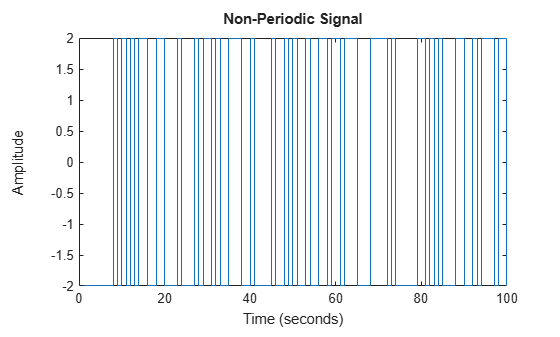The generated signal is a nonperiodic PRBS of length 100 that switches between -2 and 2.

Specify that the pseudorandom binary input signal (PRBS) switches between -2 and 2.

`Range = [-2,2];`

Specify the clock period of the signal as 1 sample. That is, the signal value can change at each time step. For PRBS signals, the clock period is specified in `Band` = [0 B], where `B` is the inverse of the required clock period.

`Band = [0 1];`

Generate a single-channel, periodic PRBS with a period of 100 samples and 3 periods in the signal.

`u1 = idinput([100,1,3],'prbs',Band,Range);`
```Warning: The period of the PRBS signal was changed to 63. Accordingly, the length of the generated signal will be 189. ```

A PRBS is inherently periodic with a maximum period length of ${2}^{n}-1$, where integer n is the order of the PRBS. If the period you specify is not equal to a maximum length PRBS, the software adjusts the period of the generated signal to obtain an integer number of maximum length PRBS, and issues a warning. For more information about maximum length PRBS, see Pseudorandom Binary Signals. In this example, the desired period, 100, is not equal to a maximum length PRBS, thus the software instead generates a maximum length PRBS of order `n = floor(log2(Period)) = 6`. Thus, the period of the PRBS signal is 63 ( = ${2}^{6}-1$), and the length of the generated signal is 189 (= `NumPeriod`*63). This result is indicated in the generated warning.

Create an `iddata` object from the generated signal, and plot the signal. Specify the period of the signal as 63 samples.

```u1 = iddata([],u1,1,'Period',63); plot(u1) title('Periodic Signal')```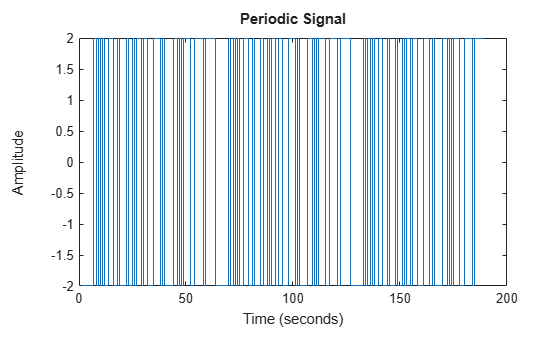The generated signal is a periodic PRBS with three periods.

Generate periodic and nonperiodic pseudorandom binary input signals (PRBS) with specified clock period.

Generate a single-channel PRBS that switches between -2 and 2. Specify the clock period of the signal as 4 samples. That is, the signal has to stay constant for at least 4 consecutive samples before it can change. For PRBS signals, the clock period is specified in `Band` = [0 B], where `B` is the inverse of the required clock period.

```Range = [-2,2]; Band = [0 1/4];```

First generate a nonperiodic signal of length 100.

`u1 = idinput(100,'prbs',Band,Range);`
```Warning: The PRBS signal delivered is the 100 first values of a full sequence of length 124. ```

To understand the generated warning, first note that the code is equivalent to generating a single-channel PRBS with a 100-sample period and 1 period.

`u1 = idinput([100,1,1],'prbs',Band,Range);`

The generated PRBS signal has to remain constant for at least 4 samples before the value can change. To satisfy this requirement, the software first computes the order of the smallest possible maximum length PRBS as `n = floor(log2(Period*B)) = 4` and period ${2}^{n}-1=15$. For information about maximum length PRBS, see Pseudorandom Binary Signals. The software then stretches this PRBS such that the period of the stretched signal is $P=\left(1/B\right)\left({2}^{n}-1\right)=60$.

However, since this period is less than the specified length, 100, the software computes instead a maximum length PRBS of order `m = n+1 = 5`. The software then stretches this PRBS such that the period is now $P2=\left(1/B\right)\left({2}^{m}-1\right)=124$. The software returns the first 100 samples of this signal as `u1`. This result ensures that the generated signal is not periodic but is constant for every 4 samples.

Create an `iddata` object from the generated signal. For this example, specify the sample time as 1 second.

`u1 = iddata([],u1,1);`

Plot, and examine the signal.

```plot(u1); title('Nonperiodic Signal')```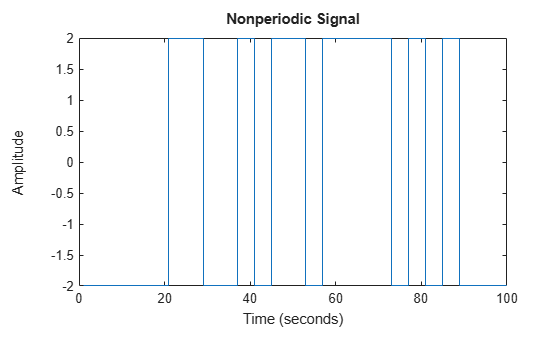The generated signal is a nonperiodic PRBS of length 100. The signal remains constant for at least 4 samples before each change in value. Thus, the signal satisfies the clock period specified in `Band`.

Now generate a periodic signal with a 100-sample period and 3 periods.

`u2 = idinput([100,1,3],'prbs',Band,Range);`
```Warning: The period of the PRBS signal was changed to 60. Accordingly, the length of the generated signal will be 180. ```

To generate a periodic signal with specified clock period, the software generates `u2` as 3 repetitions of the original stretched signal of period `P = 60`. Thus, the length of `u2` is `P*NumPeriod = 60*3 = 180`. This change in period and length of the generated signal is indicated in the generated warning.

Create an `iddata` object from the generated signal, and plot the signal. Specify the period of the signal as 60 seconds.

```u2 = iddata([],u2,1,'Period',60); plot(u2) title('Periodic Signal')```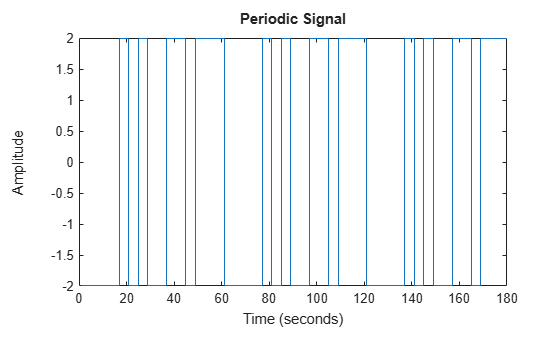The generated signal is a periodic PRBS with a 60-second period and 3 periods. The signal remains constant for at least 4 samples before each change in value. Thus, the signal satisfies the specified clock period.

You can generate a sum-of-sinusoids signal using default characteristics for the sine waves. Alternatively, you configure the number of sine waves, and the frequencies and phases of the sine waves. This example shows both approaches.

Specify that the signal has 50 samples in each period and 3 periods. Also specify that the signal amplitude range is between -1 and 1.

```Period = 50; NumPeriod = 3; Range = [-1 1];```

Specify the frequency range of the signal. For a sum-of-sinusoids signal, you specify the lower and upper frequencies of the passband in fractions of the Nyquist frequency. In this example, use the entire frequency range between 0 and Nyquist frequency.

`Band = [0 1];`

First generate the signal using default characteristics for the sine waves. By default, the software uses 10 sine waves to generate the signal. The software assigns a random phase to each sinusoid, and then changes these phases 10 times to get the smallest signal spread. The signal spread is the difference between the minimum and the maximum value of the signal over all samples.

`[u,freq] = idinput([Period 1 NumPeriod],'sine',Band,Range);`

The software returns the sum-of-sinusoids signal in `u` and the frequencies of the sinusoids in `freq`. The values in `freq` are scaled assuming that the sample time is 1 time unit. Suppose that the sample time is 0.01 hours. To retrieve the actual frequencies in rad/hours, divide the values by the sample time.

```Ts = 0.01; % Sample time in hours freq = freq/Ts; freq(1)```
```ans = 12.5664 ```

`freq(1)` is the frequency of the first sine wave. To see how the software chooses the frequencies, see the `SineData` argument description on the `idinput` reference page.

To verify that 10 sine waves were used to generate the signal, you can view the frequency content of the signal. Perform a Fourier transform of the signal, and plot the single-sided amplitude spectrum of the signal.

```ufft = fft(u); Fs = 2*pi/Ts; % Sampling frequency in rad/hour L = length(u); w = (0:L-1)*Fs/L; stem(w(1:L/2),abs(ufft(1:L/2))) % Plot until Nyquist frequency title('Single-Sided Amplitude Spectrum of u(t)') xlabel('Frequency (rad/hour)') ylabel('Amplitude')```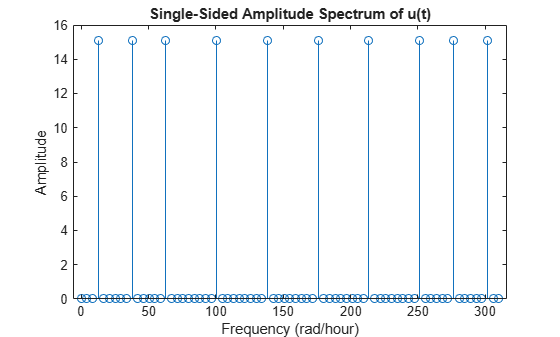The generated plot shows the frequencies of the 10 sine waves used to generate the signal. For example, the plot shows that the first sine wave has a frequency of 12.57 rad/hour, the same as `freq(1)`.

Convert the generated signal into an `iddata` object, and plot the signal. Specify the sample time as 0.01 hours.

```u = iddata([],u,Ts,'TimeUnit','hours'); plot(u)```The signal `u` is generated using 10 sinusoids and has a period of 0.5 hours and 3 periods.

Now modify the number, frequency, and phase of the sinusoids that are used to generate the sum-of-sinusoids signal. Use 12 sinusoids and try 15 different sets of phases. To set the frequencies of the sinusoids, specify `GridSkip` = 2. The software selects the frequencies of the sinusoids from the intersection of the frequency grid `2*pi*[1:GridSkip:fix(Period/2)]/Period` and the passband `pi*Band`.

```NumSinusoids = 12; NumTrials = 15; GridSkip = 2; SineData = [NumSinusoids,NumTrials,GridSkip]; u2 = idinput([Period 1 NumPeriod],'sine',Band,Range,SineData);```

Convert the generated signal into an `iddata` object, and plot the signal.

```u2 = iddata([],u2,Ts,'TimeUnit','hours'); plot(u2)```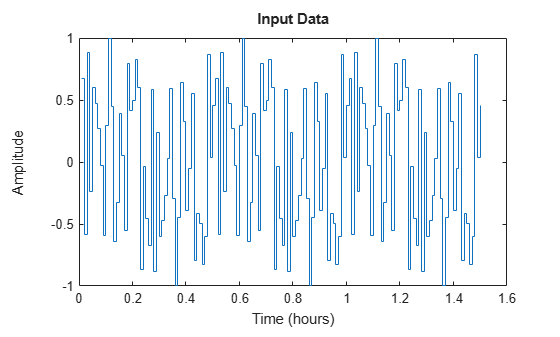The signal `u2` is generated using 12 sinusoids and has a period of 0.5 hours and 3 periods.

## Input Arguments

collapse all

Number of generated input data samples, specified as a real positive integer. For a single-channel input data, the generated input `u` has `N` rows. For an `Nu`-channel input data, `u` is returned as an `N`-by-`Nu` matrix, where each channel signal has length `N`.

Number of input channels in generated signal, specified as a real positive integer.

Number of samples in each period of generated signal, specified as a real positive integer. Use this input to specify a periodic signal. Also specify the number of periods in `NumPeriod`. Each generated input channel signal has `NumPeriod*Period` samples.

Number of periods in generated signal, specified as a real positive integer. Use this input to specify a periodic signal. Also specify the signal `Period`. Each generated input channel signal has `NumPeriod*Period` samples.

Type of generated signal, specified as one of the following values:

• `'rbs'` — Generates a random binary signal. A random binary signal is a random process that assumes only two values. You can specify these values using `Range`. To generate a band-limited signal, specify the passband in `Band`. To generate a periodic signal, specify `Period` and `NumPeriod`.

• `'rgs'` — Generates a random Gaussian signal. The generated Gaussian signal has mean μ and standard deviation σ such that [μ-σ, μ+σ] equals `Range`. To generate a band-limited Gaussian signal, specify the passband in `Band`. To generate a periodic Gaussian signal with an `n` samples period that repeats itself `m` times, specify `Period` as `n` and `NumPeriod` as `m`.

• `'prbs'`— Generates a pseudorandom binary signal (PRBS). A PRBS is a periodic, deterministic signal with white-noise-like properties that shifts between two values. You can specify these two values using `Range`. You can also specify the clock period, the minimum number of sampling intervals for which the value of the signal does not change. You specify the inverse of the clock period in `Band`.

The length of the generated signal is not always the same as what you specify. The length depends on whether you require a periodic or nonperiodic signal and also on the clock period you specify. For more information, see Pseudorandom Binary Signals.

• `'sine'`— Generates a signal that is a sum-of-sinusoids. The software selects the frequencies of the sinusoids to be equally spread over a chosen grid and assigns each sinusoid a random phase. The software then tries several random phases for each sinusoid and selects the phases that give the smallest signal spread. The signal spread is the difference between the minimum and the maximum value of the signal over all samples. The amplitude of the generated sum-of-sinusoids signal is scaled to satisfy the `Range` you specify.

You can specify the characteristics of the sine waves used to generate the signal, such as the number of sine waves and their frequency separation, in the `SineData` argument.

Frequency range of generated signal, specified as a 1-by-2 row vector containing minimum and maximum frequency values.

• If `Type` is `'rgs'`, `'rbs'`, or `'sine'` — Specify `Band` as a passband `[wlow whigh]`. Where, `wlow` and `whigh` are the lower and upper frequencies of the passband, expressed in fractions of the Nyquist frequency. For example, to generate an input with white noise characteristics, use `Band = [0 1]`.

The software achieves the frequency contents for a random Gaussian signal (`'rgs'`) using `idfilt` with an eighth-order Butterworth, noncausal filter. For generating a random binary signal (`'rbs'`), the software uses the same filter and then makes the signal binary. Thus, the frequency content in the generated random binary signal may not match the specified passband.

For `'sine'` signals, the frequencies of the sinusoids are selected to be equally spread over a chosen grid in the specified passband. For more information, see the `SineData` argument description.

• If `Type` is `'prbs'` — Specify `Band` as `[0 B]`, where `B` is the inverse of the clock period of the signal. The clock period is the minimum number of sampling intervals for which the value of the signal does not change. Thus, the generated signal is constant over intervals of length `1/B` samples. If `1/B` is not an integer, the software uses `floor(1/B)` as the clock period.

Generated input signal range, specified as a two-element row vector of the form `[umin,umax]`.

• If `Type` is `'rbs'` or `'prbs'`— The generated signal `u` has values `umin` or `umax`.

• If `Type` is `'sine'` — The generated signal `u` has values between `umin` and `umax`.

• If `Type` is `'rgs'` — The generated Gaussian signal has mean μ and standard deviation σ such that `umin` and `umax` are equal to μ-σ and μ+σ, respectively. For example, ```Range = [-1,1]``` returns a Gaussian white noise signal with zero mean and variance one.

Characterization of sinusoids used to generate a sum-of-sinusoids signal, specified as a three-element row vector `[NumSinusoids,NumTrials,GridSkip]`. Where,

• `NumSinusoids` is the number of sinusoids used to generate the signal. The default value is `10`.

• `NumTrials` is the number of different random relative phases of the sinusoids that the software tries to find the lowest signal spread. The signal spread is the difference between the minimum and the maximum value of the signal over all samples.

The maximum amplitude of the sum-of-sinusoids signal depends on the relative phases of the different sinusoids. To find the phases that give the smallest signal spread, the software tries `NumTrials` different random choices of phases to find the best phase values. For example, suppose that `NumSinusoids` is `20` and `NumTrials` is `5`. The software tries 5 different sets of relative phases for the 20 sinusoids, and selects the phases that give the smallest signal spread. The default value for `NumTrials` is `10`.

• `GridSkip` is used to characterize the frequency of the sinusoids. The software selects the frequency of the sinusoids from the intersection of the frequency grid `2*pi*[1:GridSkip:fix(Period/2)]/Period` and the pass band `pi*[Band(1) Band(2)]`. For multichannel input signals, the software uses different frequencies from this frequency grid to generate the different input channels. You can use `GridSkip` for controlling odd and even frequency multiples, for example, to detect nonlinearities of different kinds.

To extract the frequencies `freq` that are selected by the software to generate the signal, use the following syntax.

`[u,freq] = idinput(__)`

## Output Arguments

collapse all

Generated input signal, returned as a column vector of length `N` for a single-channel input or an `N`-by-`Nu` matrix for an `Nu`-channel signal. You use the generated signal to simulate the response of your system using `sim`.

You can create an `iddata` object from `u` by specifying output data as `[]`.

```u = iddata([],u); ```

In the `iddata` object, you can also specify the properties of the signal such as sample time, input names, and periodicity.

Frequencies of sine waves used for sum-of-sinusoids signal, returned as a column vector of length equal to the number of sinusoids, `NumSinusoids`. You specify `NumSinusoids` in the `SineData` argument. The frequency values are scaled assuming the sample time is 1 time unit. To retrieve the actual frequencies, divide the values by the sample time. For an example, see Generate a Sum-of-Sinusoids Signal.

For multichannel input signals, `freq` is an `Nu`-by-`NumSinusoids` matrix where the kth row contains the frequencies corresponding to the kth channel. For information about how the software selects the frequencies, see the `SineData` argument description.

collapse all

### Pseudorandom Binary Signals

A pseudorandom binary signal (PRBS) is a periodic, deterministic signal with white-noise-like properties that shifts between two values.

A PRBS is generated as:

`$u\left(t\right)=rem\left({a}_{1}u\left(t-1\right)+\dots +{a}_{n}u\left(t-n\right),2\right)$`

Here, $u\left(t-1\right),\dots u\left(t-n\right)$ is the vector of past inputs, n is the PRBS order, and `rem` denotes the remainder when $\left({a}_{1}u\left(t-1\right)+\dots {a}_{n}u\left(t-n\right)\right)$ is divided by 2. Thus, a PRBS can only take the values 0 and 1. The software scales these values according to the `Range` you specify. In addition, the vector of past inputs $u\left(t-1\right),\dots u\left(t-n\right)$ can only take `2n` values. Out of these values, the state with all zeros is ignored because it will result in future signals equal to zero. Thus, a PRBS is an inherently periodic signal with a maximum period length of `2n-1`. The following table lists the maximum length possible for different orders n of the PRBS.

Order nMaximum length PRBS (`2n-1`)
23
37
415
531
663
7127
324294967295

### Note

The software does not generate signals with period greater than `232-1`.

#### Length of Generated PRBS

Since PRBS are inherently periodic, the length and period of the generated signal depends on the clock period that you specify and whether you require a periodic or nonperiodic signal. The clock period is the minimum number of sampling intervals for which the value of the signal does not change. You specify the clock period in `Band`.

Clock period = 1 sample (```Band = [0 B] = [0 1]```):

• To generate a nonperiodic signal of length `N`, (`NumPeriod` = 1), the software first computes a maximum length PRBS with a period greater than `N`. The software then returns the first `N` samples of the PRBS as `u`. This action ensures that `u` is not periodic. For example, if `N` is 100, the software creates a maximum length PRBS of period 127 (order 7), and returns the first 100 samples as `u`.

For an example, see Generate a Nonperiodic Pseudorandom Binary Input Signal.

• To generate a periodic signal (`NumPeriod` > 1), the software adjusts the period of the signal to obtain an integer number of maximum length PRBS. To do so, the software computes a PRBS of order `n = floor(log2(Period))` and period ```P = 2n-1```. The signal `u` is then generated as `NumPeriod` repetitions of this PRBS signal of period `P`. Thus, the length of `u` is `P*NumPeriod`.

For an example, see Generate a Periodic Pseudorandom Binary Input Signal.

In the multiple-input channel case, the signals are maximally shifted. That is, the overlap between the different inputs is minimized. This means `Period/NumPeriod` is an upper bound for the model orders that you can estimate using such a signal.

Clock period > 1 sample (```Band = [0 B], where B<1```):

The generated signal has to remain constant for at least `1/B` samples. To satisfy this requirement, the software first computes the order of the smallest possible maximum length PRBS as `n = floor(log2(Period*B))` and period `2n-1`. The software then stretches the PRBS such that period of the stretched signal is ```P = B-1(2n-1)```.

• To generate a nonperiodic signal of length `N` , if the period `P` of the stretched signal is greater than or equal to `N`, the software returns the first `N` samples of the stretched signal as `u`. This ensures that `u` is nonperiodic but constant for every `1/B` samples. Note that for a nonperiodic signal, `Period` is equal to `N`.

If the period `P` is less than `N`, the software computes instead a maximum length PRBS of order ```n2 = n+1```. The software then stretches this PRBS such that the period is now `P2 = B-1(2n2-1)`. The software then returns the first `N` samples of this signal as `u`.

• To generate a periodic signal, the software generates `u` as `NumPeriod` repetitions of the stretched signal of period `P`. Thus, the length of `u` is `P*NumPeriod`.

For an example, see Generate Pseudorandom Binary Input Signal with Specified Clock Period.

 Söderström, T. and P. Stoica., Chapter C5.3 in System Identification, Prentice Hall, 1989.

 Ljung, L., Section 13.3 in System Identification: Theory for the User, Prentice Hall PTR, 1999.

##### SupportGet trial now